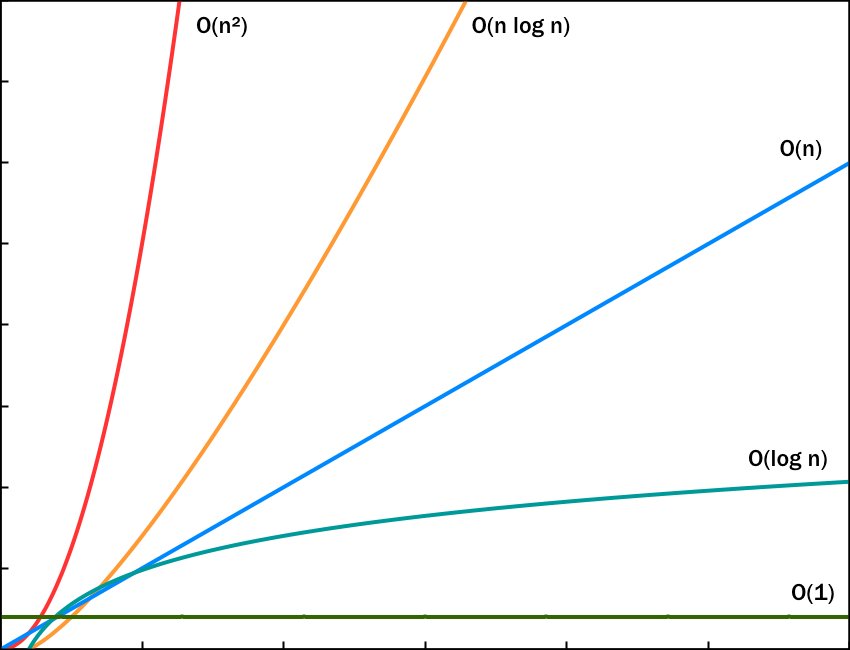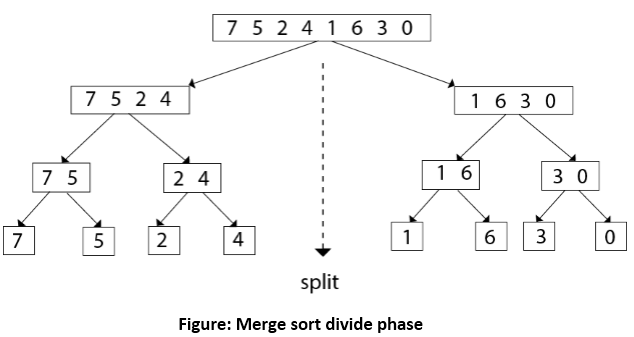### Introduction

Following the insertion sort algorithm which we have implemented in the previous post, it was only natural to follow with the implementation of merge sort.

Compared with the insertion sort which has a time complexity of O(n2), the merge sort is more efficient, with a time complexity of O(n*log(n)). This means that it will be able to handle an increasing number of elements faster. See the graph below for a visual comparison.The strategy used by this algorithm is called divide and conquer, which means that we split our problem in smaller chunks, which makes it easier to solve.

There are two main steps in performing this type of a sort. First we have to divide the array and then merge the elements. Both of these will happen recursively. So every time we merge, we have two sorted arrays, which we have to combine into one. This is done easily by popping the smallest element of one of the two arrays until one of them is depleted and then appending the rest.

Visually, it would look something like this.### Implementation

The code for merge sort is not as straight forward as for the insertion sort but it makes sense if analysed carefully.

``````package mergesort

// This is the only exported function which will take a slice as an input
// and return a different slice with the original integers sorted
func Sort(input []int) []int {
// making a new slice and copying the contents of the input
// into it
// this slice is going to be subsequently mutated so that
// we get the desired order of elements
sorted := make([]int, len(input))
copy(sorted, input)

// calling the private "subSort" function which can sort a
// sub-slice, by taking two more arguments: the start and
// end index
subSort(sorted, 0, len(input)-1)
return sorted
}

// this function is going to call itself recursively with the left
// and the right halves of the input slice (the divide)
// then call the "merge" function (the conquest)
func subSort(sorted []int, leftStart int, rightEnd int) {
// stop the recursion if there is nothing to divide
if leftStart >= rightEnd {
return
}
// calculating the middle element so that we can divide our
// slice
middle := (leftStart + rightEnd) / 2
// calling itself recursively with both halves
subSort(sorted, leftStart, middle)
subSort(sorted, middle+1, rightEnd)
// merging the two sorted halves
merge(sorted, leftStart, rightEnd)
}

func merge(sorted []int, leftStart int, rightEnd int) {
// creating a temporary slice, as we can't easily do it in place
temp := make([]int, len(sorted))
copy(temp, sorted)

// the end of the left sub-slice will end in the middle
leftEnd := (leftStart + rightEnd) / 2
// the start of the right slice will be right after the left ends
rightStart := leftEnd + 1

left := leftStart
right := rightStart
index := leftStart

// this is the loop where the actual sorting happens
// we iterate until either the left or the right sub-slice
// runs out of elements
for left <= leftEnd && right <= rightEnd {
// here we start adding elements to the temporary
// slice we created above
// we choose the smaller element every time
if sorted[left] < sorted[right] {
temp[index] = sorted[left]
left++
} else {
temp[index] = sorted[right]
right++
}
index++
}

// here we append to the temporary slice the remaining elements
// that were not picked in the loop above
// first we do it for the left and the for the right sub-slice
// one of them will not contain any remaining elements
// so will make no changes
copy(temp[index:rightEnd+1], sorted[right:rightEnd+1])
copy(temp[index:rightEnd+1], sorted[left:leftEnd+1])
// finally we store the sorted numbers from the temporary slice
// into our sorted slice
copy(sorted, temp)
}

``````

### Source code

https://github.com/dorin131/go-algorithms